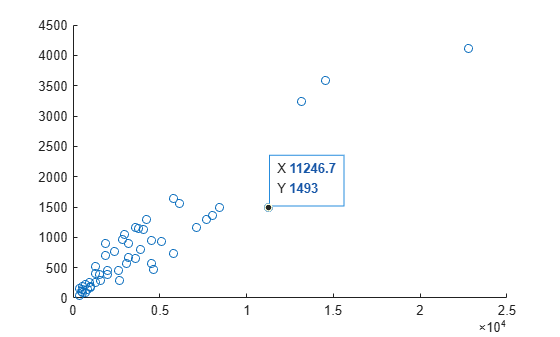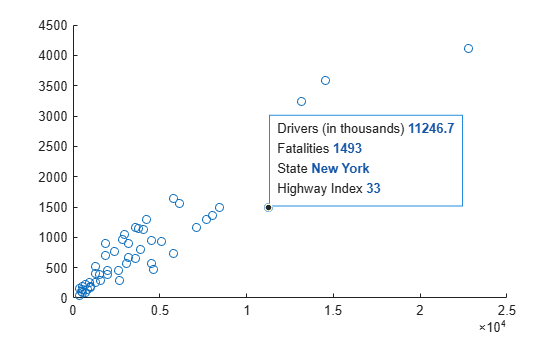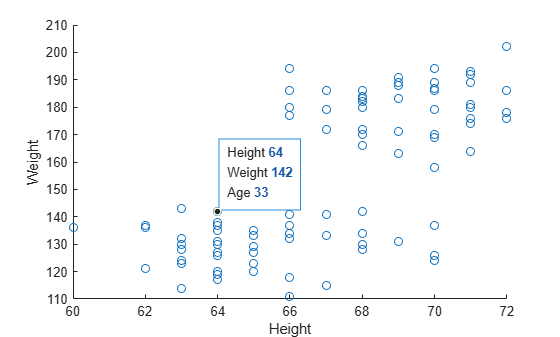## Create Custom Data Tips

Data tips appear when you hover over a data point. By default, the data tips include the data specified during chart creation that correspond to the individual data point. However, for some types of charts, you can customize the information that appears in the data tip, such as changing the labels or adding new rows.

Charts that support these customizations have a `DataTipTemplate` property, for example, `Line` objects created with the `plot` function.

### Change Labels and Add Row

Modify the contents of data tips on a scatter plot. First, load sample accident data and create the scatter plot. Then, create a data tip interactively or by using the `datatip` function. By default, data tips show the coordinates of the data point.

```load("accidents.mat","hwydata","statelabel","hwyidx") s = scatter(hwydata(:,5),hwydata(:,4)); dt = datatip(s,11246.7,1493);```Change the data tip labels from `X` and `Y` to `Drivers (in thousands)` and `Fatalities` by accessing the `DataTipTemplate` property of the plotted object and setting the `Label` property for each row.

```s.DataTipTemplate.DataTipRows(1).Label = "Drivers (in thousands)"; s.DataTipTemplate.DataTipRows(2).Label = "Fatalities"; ```

Add new rows to the data tip. For the labels, use `State` and `Highway Index`. For the values, use the state names and highway indexes contained in the `statelabel` and `hwyidx` variables in your workspace.

```dtRows = [dataTipTextRow("State",statelabel),... dataTipTextRow("Highway Index",hwyidx)]; s.DataTipTemplate.DataTipRows(end+1:end+2) = dtRows;```### Show Table Values in Data Tips

Modify the contents of data tips for a scatter plot to include additional values from a table. First, create a table from a sample spreadsheet of patient data. Plot the data. Then, create a data tip interactively or by using the `datatip` function.

```tbl = readtable("patients.xls"); s = scatter(tbl,"Height","Weight"); dt = datatip(s,64,142);```

Add a new row to the data tip that uses the label `Age` and shows the values from the Age column of the table.

```row = dataTipTextRow("Age",tbl.Age); s.DataTipTemplate.DataTipRows(end+1) = row;```